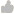Support Center > Knowledge base> Article: What does the Index Score for each Training Program mean? How was the score calculated?

What does the Index Score for each Training Program mean? How was the score calculated?

Article ID: 98580Question
What does the Index Score for each Training Program mean? How was the score calculated?